# Erlang - Shell

The Erlang shell is used for testing of expressions. Hence, testing can be carried out in the shell very easily before it actually gets tested in the application itself.

The following example showcases how the addition expression can be used in the shell. What needs to be noted here is that the expression needs to end with the dot (.) delimiter.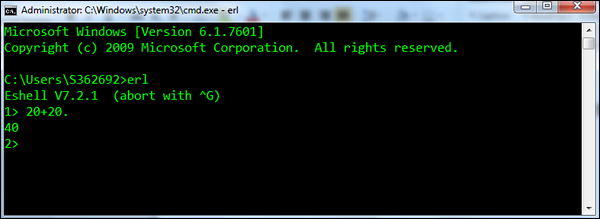After the command is executed, the shell prints out another prompt, this time for Command Number 2 (because the command number increases each time a new command is entered).

The following functions are the most common one’s used in the Erlang shell.

• b() − Prints the current variable bindings.

• Syntax − b().

• For example − Following is an example of how the function is used. First a variable called Str is defined, which has the value abcd. Then b() is used to display all the binded variables.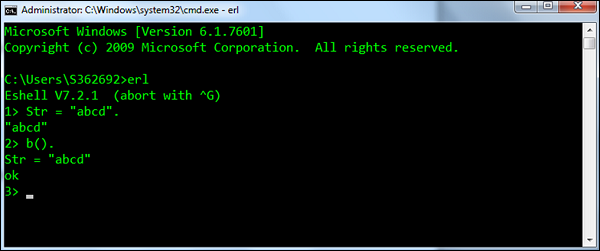• f() − Removes all current variable bindings.

• Syntax − f().

• For example − Following is an example of how the function is used. First a variable called Str is defined which has the value abcd. The f() is then used to remove the Str variable binding. The b() is then called to ensure the binding has been successfully removed.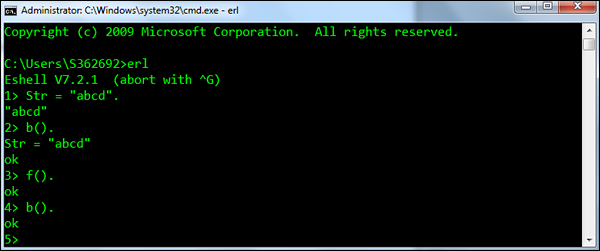• f(x) − Removes the binding for a particular variable.

• Syntax − f(x). Where, x – is the variable for which the binding needs to be removed.

• For example − Following is an example of how the function is used. First a variable called Str and Str1 are defined. The f(Str) is then used to remove the Str variable binding. The b() is then called to ensure the binding has been successfully removed.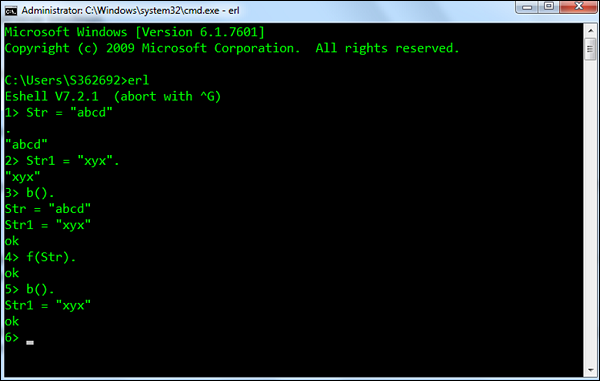• h() − Prints the history list of all the commands executed in the shell.

• Syntax − h().

• For example − An example of the h() command, which prints the history of commands executed in the shell is shown in the following screenshot.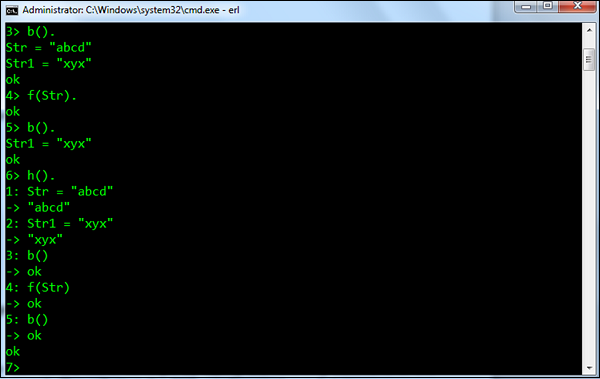• history(N) − Sets the number of previous commands to keep in the history list to N. The previous number is returned. The default number is 20.

• Syntax − history(N). Where, N – is the number to which the command history list needs to be limited to.

• For example − An example of the history(N) command is shown in the following screenshot.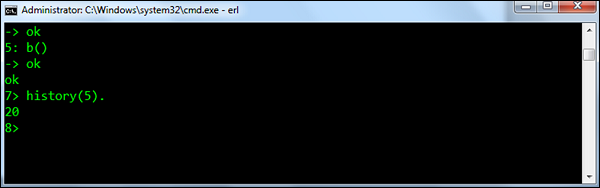• e(N) − Repeats the command N, if N is positive. If it is negative, the Nth previous command is repeated (i.e., e(-1) repeats the previous command).

• Syntax − e(N). Where, N – is the command at the Nth position in the list.

• For example − An example of the e(N) command is shown below. Since we have executed the e(-1) command, it will execute the previous command which was history(5).